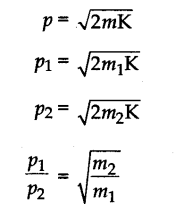# (a) Is it possible that a body be in accelerated motion under the action of a force, yet no work is being done by the force? Explain with an example. (b) Two bodies of masses m1 and m2 have equal kinetic energies.What is the ratio of their linear momenta?

(a) Yes, it is possible in the case of a body moving in a circular path with a speed v. The body has a centripetal acceleration directed along the radius of the circular path. The displacement is, however, tangential to the radius i.e., 0 (theta) = 90°. Thus, work done
W = FS cos 90° = 0.

(b) We know that the relation between kinetic energy K and momentum p asRelated questions

3 months ago
1

3 months ago
1

3 months ago
1

3 months ago
1

3 months ago
1

3 months ago
1

3 months ago
1

3 months ago